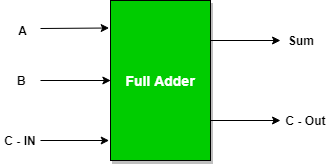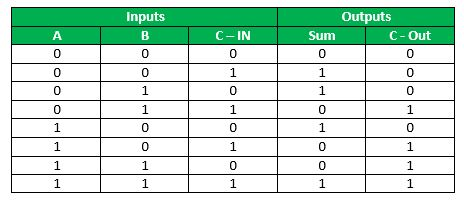# Python program to implement Full Adder

• Difficulty Level : Basic
• Last Updated : 09 Jun, 2021

Prerequisite : Full Adder in Digital Logic
Given three inputs of Full Adder A, B,C-IN. The task is to implement the Full Adder circuit and Print output i.e sum and C-Out of three inputs.

Full Adder : A Full Adder is a logical circuit that performs an addition operation on three one-bit binary numbers. The full adder produces a sum of the three inputs and carry value.Logical Expression :

```SUM  =  C-IN  XOR  ( A XOR B )
C-0UT= A B + B C-IN + A C-IN```

Truth Table :Examples :

```Input : 0 1 1
Output: Sum=0, C-Out=1```

According to logical expression Sum= C-IN XOR (A XOR B )  i.e 1 XOR (0 XOR 1) =0 , C-Out= A B + B C-IN + A C-IN  i.e.,  0 AND 1 + 1 AND 1 + 0 AND 1 = 1

```Input : 1 0 0
Output: Sum=1, C-Out=0```

Approach :

• We take three inputs A ,B and C-in .
• Applying C-IN XOR (A XOR B ) gives the value of sum
• Applying  A B + B C-IN + A C-IN gives the value of C-Out

Below is the implementation :

## Python3

 `# python program to implement full adder` `# Function to print sum and C-Out``def` `getResult(A, B, C):` `    ``# Calculating value of sum``    ``Sum` `=` `C ^ (A ^ B)``    ``C``    ``# Calculating value of C-Out``    ``C_Out ``=` `Bin``&(``not``(A ^ B))| ``not``(A)&B` `    ``# printing the values``    ``print``(``"Sum = "``, ``Sum``)``    ``print``(``"C-Out = "``, C_Out)`  `# Driver code``A ``=` `0``B ``=` `0``C ``=` `1``# passing three inputs of fulladder as arguments to get result function``getResult(A, B, C)`

Output :

```Sum =  1
C-Out =  0```
My Personal Notes arrow_drop_up